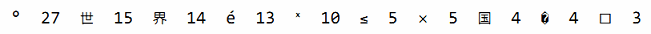## 4.3. Map

ages := make(map[string]int) // mapping from strings to ints


ages := map[string]int{
"alice":   31,
"charlie": 34,
}


ages := make(map[string]int)
ages["alice"] = 31
ages["charlie"] = 34


Map中的元素通過key對應的下標語法訪問：

ages["alice"] = 32
fmt.Println(ages["alice"]) // "32"


delete(ages, "alice") // remove element ages["alice"]


ages["bob"] = ages["bob"] + 1 // happy birthday!


ages["bob"] += 1


ages["bob"]++


_ = &ages["bob"] // compile error: cannot take address of map element


for name, age := range ages {
fmt.Printf("%s\t%d\n", name, age)
}


Map的迭代順序是不確定的，併且不同的哈希函數實現可能導致不同的遍歷順序。在實踐中，遍歷的順序是隨機的，每一次遍歷的順序都不相同。這是故意的，每次都使用隨機的遍歷順序可以強製要求程序不會依賴具體的哈希函數實現。如果要按順序遍歷key/value對，我們必鬚顯式地對key進行排序，可以使用sort包的Strings函數對字符串slice進行排序。下面是常見的處理方式：

import "sort"

var names []string
for name := range ages {
names = append(names, name)
}
sort.Strings(names)
for _, name := range names {
fmt.Printf("%s\t%d\n", name, ages[name])
}


names := make([]string, 0, len(ages))


map類型的零值是nil，也就是沒有引用任何哈希表。

var ages map[string]int
fmt.Println(ages == nil)    // "true"
fmt.Println(len(ages) == 0) // "true"


map上的大部分操作，包括査找、刪除、len和range循環都可以安全工作在nil值的map上，它們的行爲和一個空的map類似。但是向一個nil值的map存入元素將導致一個panic異常：

ages["carol"] = 21 // panic: assignment to entry in nil map


age, ok := ages["bob"]
if !ok { /* "bob" is not a key in this map; age == 0. */ }


if age, ok := ages["bob"]; !ok { /* ... */ }


func equal(x, y map[string]int) bool {
if len(x) != len(y) {
return false
}
for k, xv := range x {
if yv, ok := y[k]; !ok || yv != xv {
return false
}
}
return true
}


// True if equal is written incorrectly.
equal(map[string]int{"A": 0}, map[string]int{"B": 42})


Go語言中併沒有提供一個set類型，但是map中的key也是不相同的，可以用map實現類似set的功能。爲了説明這一點，下面的dedup程序讀取多行輸入，但是隻打印第一次出現的行。（它是1.3節中出現的dup程序的變體。）dedup程序通過map來表示所有的輸入行所對應的set集合，以確保已經在集合存在的行不會被重複打印。

gopl.io/ch4/dedup

func main() {
seen := make(map[string]bool) // a set of strings
input := bufio.NewScanner(os.Stdin)
for input.Scan() {
line := input.Text()
if !seen[line] {
seen[line] = true
fmt.Println(line)
}
}

if err := input.Err(); err != nil {
fmt.Fprintf(os.Stderr, "dedup: %v\n", err)
os.Exit(1)
}
}


Go程序員將這種忽略value的map當作一個字符串集合，併非所有map[string]bool類型value都是無關緊要的；有一些則可能會同時包含tue和false的值。

var m = make(map[string]int)

func k(list []string) string { return fmt.Sprintf("%q", list) }

func Add(list []string)       { m[k(list)]++ }
func Count(list []string) int { return m[k(list)] }


gopl.io/ch4/charcount

// Charcount computes counts of Unicode characters.
package main

import (
"bufio"
"fmt"
"io"
"os"
"unicode"
"unicode/utf8"
)

func main() {
counts := make(map[rune]int)    // counts of Unicode characters
var utflen [utf8.UTFMax + 1]int // count of lengths of UTF-8 encodings
invalid := 0                    // count of invalid UTF-8 characters

for {
r, n, err := in.ReadRune() // returns rune, nbytes, error
if err == io.EOF {
break
}
if err != nil {
fmt.Fprintf(os.Stderr, "charcount: %v\n", err)
os.Exit(1)
}
if r == unicode.ReplacementChar && n == 1 {
invalid++
continue
}
counts[r]++
utflen[n]++
}
fmt.Printf("rune\tcount\n")
for c, n := range counts {
fmt.Printf("%q\t%d\n", c, n)
}
fmt.Print("\nlen\tcount\n")
for i, n := range utflen {
if i > 0 {
fmt.Printf("%d\t%d\n", i, n)
}
}
if invalid > 0 {
fmt.Printf("\n%d invalid UTF-8 characters\n", invalid)
}
}


charcount程序同時打印不同UTF-8編碼長度的字符數目。對此，map併不是一個合適的數據結構；因爲UTF-8編碼的長度總是從1到utf8.UTFMax（最大是4個字節），使用數組將更有效。len count
1   765391
2   60
3   70
4   0


Map的value類型也可以是一個聚合類型，比如是一個map或slice。在下面的代碼中，圖graph的key類型是一個字符串，value類型map[string]bool代表一個字符串集合。從概念上將，graph將一個字符串類型的key映射到一組相關的字符串集合，它們指向新的graph的key。

gopl.io/ch4/graph

var graph = make(map[string]map[string]bool)

edges := graph[from]
if edges == nil {
edges = make(map[string]bool)
graph[from] = edges
}
edges[to] = true
}

func hasEdge(from, to string) bool {
return graph[from][to]
}